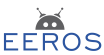#Real-Time Robotics Framework

### Sidebar

tools:matrix:start

# Vectors and Matrices

The blocks in the control system are connected among each other with the aid of signals. As described in Signals they carry a timestamp and a value. The value could be a simple type such as an integer or float. Quite often it will consist of a vector or a matrix. A three dimensional vector of type double can be declared and initialized as follows:

  Vector<3, double> v;
v << 1.5, -2, 0;

The declaration could be simplified to

  Vector3<> v;
v << 1.5, -2, 0;

as Vector3 is a predefined type with three dimensions and the default element type is double. A matrix of 3 times 3 with element type int could be defined as

  Matrix<3, 3, int> m;
m << 1, 4, 7,
2, 5, 8,
3, 6, 9;

## Accessing Elements

You can access rows, columns or single elements of matrices with the following methods:

m.get(0,0);  // returns element, 1
m.getRow(1);  // returns row, [2,5,8]
m.getCol(0);  // returns column, [1,2,3]'
m.getSubMatrix<2,2>(0,1);   // returns matrix [[4,5][7,8]]

Single elements, rows or columns can be written with the methods set(), setRow(), or setCol().

The operators () and [] work as well as can be seen by the following example

m(3);   // returns element or sets element with index 3
m;   // returns element or sets element with index 3
m(2,1);   // returns element or sets element with index 7